##### Signals and Systems For Dummies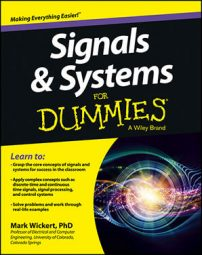Continuous-time signals and systems never take a break. When a circuit is wired up, a signal is there for the taking, and the system begins working — and doesn’t stop. Keep in mind that the term signal is used here loosely; any one specific signal may come and go, but a signal is always present at each and every time instant imaginable in a continuous-time system.

## Continuous-time signals

Continuous signals function according to time t. A sinusoidal function of time is one of the most basic signals. The mathematical model for a sinusoid signal is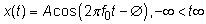where A is the signal amplitude, f0 is the signal frequency, and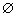is the signal phase shift. The independent variable is time t. If you’re curious about the first peak of x (t) occurring at 3/16, notice that this occurs when the argument of the cosine is 0 — that is,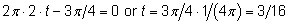Check out the plot of a sinusoid signal.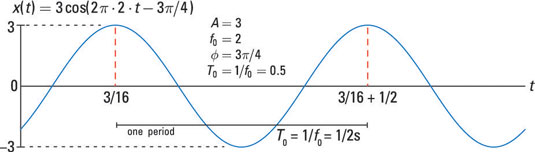The amplitude of this signal is 3, the frequency is 2 Hz, and the phase shift is 3π/4 rad.

## Continuous-time systems

Systems operate on signals. In mathematical terms, a system is a function or operator, T{}, that maps the input signal x(t) to output signal y(t) = T{x(t)}.

An example of a continuous-time system is the electronic circuits in an amplifier, which has gain 5 and level shift 2: y(t) = T{x[n]} = 5x(t) + 2.

See a block diagram representation of this simple system: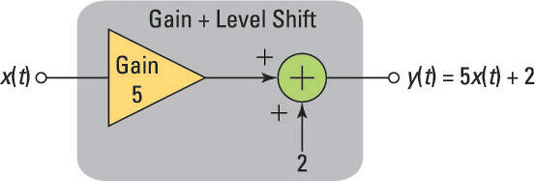Building an amplifier that corresponds to this mathematical model is another matter entirely. You can create a simple electronic circuit, but it will have limitations that the math model doesn’t have. It’s up to you, as an electronic engineer, to refine the model to accurately reflect the level of detail needed to assess overall performance of a design candidate.